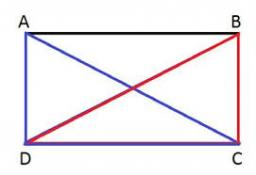# Circumference 4003

Calculate the diagonal length of a rectangle whose length is 3 cm greater than its width and whose circumference is 18 centimeters.

u =  6.7082 cm

### Step-by-step explanation:Did you find an error or inaccuracy? Feel free to write us. Thank you!

Tips for related online calculators
Do you have a linear equation or system of equations and looking for its solution? Or do you have a quadratic equation?
The Pythagorean theorem is the base for the right triangle calculator.

#### Grade of the word problem:

We encourage you to watch this tutorial video on this math problem: